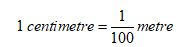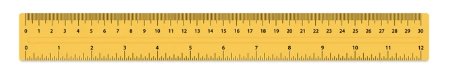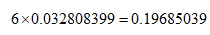×## Introduction

The centimeter unit is derived from the meter unit. The word "centi" means one-hundredth and Thus,Typically, centimeters are used to measure small distances, such as 10 cm or 12 cm.

The meter is also a unit of distance or length, just like the foot, which is equal to 30.48 cm. Similarly, an even smaller unit of distance is the millimeter, which is one-tenth of a centimeter, with one unit equal to 0.1 cm. Another unit you might be familiar with is the inch, one unit of which equals 2.54 cm.

## Conventional tools of measurement

Here are a few units that are commonly used to measure distance:

Inch: The inch is a unit of measurement that is slightly larger than the centimeter and commonly used to measure clothes for cutting, paper sizes, and other similar dimensions. It equals 2.54 cm.

Foot: The foot is another unit of length that is used in countries where the imperial system of measurement is used. One foot equals 12 inches or 30.48 cm and it is generally used in measuring people’s heights.

Cubit: The Cubit is a unit of measurement that is based on the length of the forearm, typically measured from the tip of the middle finger to the elbow. It was widely used by ancient civilizations like the Egyptians and Mesopotamians. Archaeological evidence of cubit sticks has been found in the remnants of ancient Egyptian civilization. A cubit stick is usually 20 inches (50 cm) long, and is divided into seven palms, with each palm consisting of four fingers.

Miles: Miles are used in the imperial system and most used in the United States. They are approximately equal to 1.6 km.

## Centimeter Scale

A centimeter scale is a measuring scale that typically has both centimeter and inch measurements marked on it at regular intervals. The top portion of the scale is marked in centimeters, while the bottom portion is marked in inches, as shown in the figure below.Centimeter scale

We commonly use the centimeter scale for measuring small distances, such as when creating diagrams in a notebook. In mathematics, the most commonly used measuring tool is the ruler, which is used to measure small objects like books, pencils, and bottles. Rulers are typically available in two sizes, 6 inches and 12 inches, and are marked in inches and centimeters.

Similarly, another tool of measurement is the yardstick that measures in meters and centimeters and is used for larger objects. For instance, it could be used to measure the length of a table or the width of a bag.

## Centimeter Chart

Simply speaking, a centimeter chart lists various units of length and shows how we can interconvert between these units and the centimeter. Generally, these units can include meters, inches, feet, yards, etc.

## Centimeter to Meters

As previously stated, the centimeter unit is derived from the meter unit and the word centi means one-hundredth. Thus, there are 100 cm in 1 m. In the international system of units, the meter unit is defined explicitly, while the centimeter is based on the meter instead of having an explicit definition. The definition of meter is given below:

A meter is a unit of length defined as the distance travelled by light in 1/299792458

of a second. Therefore,

1 cm = 0.01 m

1m = 100 cm

In the table given below, you can see a few example conversions between centimeters and meters:

 Centimeters Meters 1 cm 0.01 m 3 cm 0.03 m 4 cm 0.04 m 8 cm 0.08 m 40 cm 0.4 m 100 cm 1 m 500 cm 5 m 1000 cm 10 m

## Centimeter to Feet

The foot is another commonly used unit of measurement in the imperial system and is equal to 12 inches. 1 inch itself equals 2.54 cm and thus, to convert from cm to foot, we must multiply the value by 0.0328084.

Example: 6 cm is equal toCentimeters Feet 1 cm 0.038084 ft. 3 cm 0.09843 ft. 5 cm 0.016404 ft. 40 cm 1.131234 ft. 50 cm 1.64042 ft. 100 cm 3.28084 ft.

## Centimeter to Inch

Inch is also a unit of distance commonly used where the imperial system of measurement is followed. It is also used to measure clothes, paper sizes, and other similar dimensions. 1 inch equals 2.54 cm and some example conversions are given below:

 Centimeters Inches 3 cm 1.1811 in 4 cm 1.5748 in 6 cm 2.3622 in 7 cm 2.7559 in 10 cm 3.9370 in

## Centimeter to Millimeters

For measuring distances even smaller the centimeter, one can use the millimeter unit, which is equal to one-tenth of a centimeter. That is, 10 mm = 1 cm. Look at a few example conversions below:

 Centimeters Millimeters 2 cm 20 mm 4 cm 40 mm 5 cm 50 mm 10 cm 100 mm 100 cm 1000 mm 10000 cm 100000 mm

## Summary

This article discussed conversion between various units of length. A summary of the conversion factors is given below:

100 cm = 1 m, or 1 cm = 0.01 m

1 cm = 0.0328084 ft., or 1 ft. = 30.48 cm

1 cm = 0.3937 in, or 1 in = 2.54 cm

1 cm = 10 mm, or 1 mm = 0.1 cm

1. How do we convert between centimeters and meters?

Centimeters and meters have a relation of 100. That is, 1 m = 100 cm. Thus, to convert from cm to m, divide the value by 100.

2. How to convert centimeters to feet?

We know that 1 cm equals 0.0328084 feet and thus, multiplying the given value with 0.0328084 will give us the measurement in feet.

3. What do you mean by centimeter chart?

Using a centimeter chart, we can easily convert between different units of length. A centimeter chart lists various such units and their conversion factors with a centimeter.

4. How to convert centimeters to inches?

An inch is approximately equal to 2.54 cm and thus, we can divide by 2.54 or multiply by 0. 393700787 to get the answer in inches.

#### Recent Posts